# PSEB 5th Class Maths Solutions Chapter 7 Geometry Ex 7.2

Punjab State Board PSEB 5th Class Maths Book Solutions Chapter 7 Geometry Ex 7.2 Textbook Exercise Questions and Answers.

## PSEB Solutions for Class 5 Maths Chapter 7 Geometry Ex 7.2

1. Measure the following angles using protractor:

Question 1.Solution:
70°

Question 2.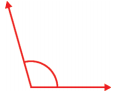Solution:
105°Question 3.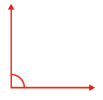Solution:
90°

Question 4.Solution:
130°

Question 5.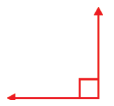Solution:
90°Question 6.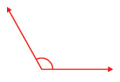Solution:
115°

Question 7.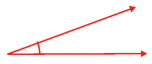Solution:
20°

Question 8.Solution:
50°

Question 9.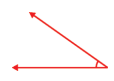Solution:
35°Question 10.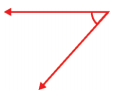Solution:
50°

2. Draw the following angles by using a protractor:

Question 1.
15°
Solution: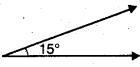Question 2.
40°
Solution: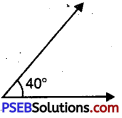Question 3.
42°
Solution: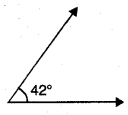Question 4.
53°
Solution: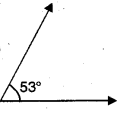Question 5.
65°
Solution: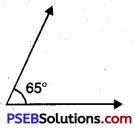Question 6.
75°
Solution: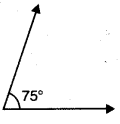Question 7.
90°
Solution: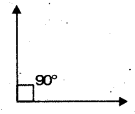Question 8.
110°
Solution: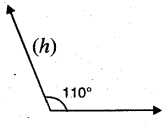Question 9.
117°
Solution: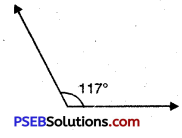Question 10.
135°
Solution: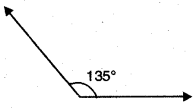Question 11.
157°
Solution: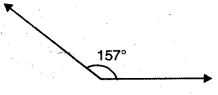Question 12.
180°
Solution: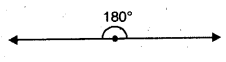3. Pick out the acute angle, obtuse angle and right angle from the following :

Question 1.
35°
Solution:
Acute angle

Question 2.
89°
Solution:
Acute angle

Question 3.
120°
Solution:
Obtuse angleQuestion 4.
100°
Solution:
Obtuse angle

Question 5.
96°
Solution:
Obtuse angle

Question 6.
74°
Solution:
Acute angle

Question 7.
62°
Solution:
Acute angleQuestion 8.
166°.
Solution:
Obtuse angle.

4. Fill in the blanks :

Question 1.
An angle between 0° and 90° is called ………………
Solution:
Acute angle

Question 2.
175° angle is ……………… angle.
Solution:
Obtuse

Question 3.
The hands of a clock make an angle of ……………….. at 3 a.m.
Solution:
right angle

Question 4.
Measurements of an angle between North and South direction is ………………….
Solution:
180°Question 5.
An acute angle is ……………….. than right angle.
Sol.
smaller.

5. Tick the True and False :

Question 1.
Measurement of right angle is 90°.
Solution:
True

Question 2.
Right angle is greater than acute angle but smaller than obtuse angle.
Solution:
True

Question 3.
On the Internal and External scale of protractor, measurements are written up to 90°.
Solution:
FalseQuestion 4.
85° is a right angle.
Solution:
False

Question 5.
115° is an obtuse angle.
Solution:
True

Question 6.
90° is an acute angle.
Solution:
False